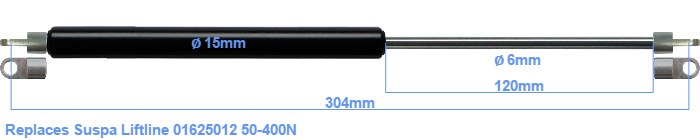# Replacement for Suspa Liftline 01625012 50-400N

£26.11 (excl. VAT)

Replacement gas spring for the Suspa Liftline 01625012 50-400 Newton. The eye has a thickness of 3mm and a hole diameter of 6.1mm. Brand: Hahn.

Please note:
The eye with the thickness of 3mm of this replacement gas spring can be shorter than the original. Note that this part of the eye doesn't get in the way.
 Force Choose an option50 Newton70 Newton75 Newton90 Newton100 Newton110 Newton125 Newton130 Newton150 Newton170 Newton175 Newton190 Newton200 Newton210 Newton225 Newton230 Newton250 Newton270 Newton275 Newton290 Newton300 Newton310 Newton325 Newton330 Newton350 Newton370 Newton375 Newton390 Newton400 NewtonClear
This gas spring is also known as 16-1 016 25012, 16-1-157,5-125-A17-B17.